# 人工智能控制

==目录==

[TOC]

## 知识表示

### 知识

• 概念: 知识反应了客观世界中事物之间的关系，不同事物或者相同事物之间的不同关系形成了不同的知识
• 特性： 知识具有相对正确性、不正确性、可表示性、可利用性
• 知识表示：将人类知识形式化或者模型化。知识表示是对知识的一种描述，或者说是一组约定，一种计算机可以接受的用于描述知识的数据结构

### 一阶谓词逻辑表示法

#### 概念

• 命题：非真即假的陈述句（一个命题在不同条件下真值可能改变）

• 原子命题：简单陈述句表达的命题称为简单命题或原子命题。

• 谓词：一般形式；$P(x_1,x_2,\dots,x_n)$,其中$P$是谓词名，$x_1,x_2,\dots,x_n$是个体

• 个体：可以是常量、变量、函数、谓词

• 谓词公式连接词：否定 $\neg$ 、析取 $\vee$ 、合取 $\wedge$ 、蕴含 $\rightarrow$ 、等价 $\leftrightarrow$

• 量词：全称量词 $\forall$ 、存在量词 $\exists$ ，（出现顺序将影响命题含义）

• 谓词公式：原子谓词公式的有限步套娃缝合

• 连接词和量词的优先级是如上出现的顺序由高到低

否定 $\neg$ 、析取 $\vee$ 、合取 $\wedge$ 、蕴含 $\rightarrow$ 、等价 $\leftrightarrow$

• 量词辖域：位于量词后面的单个谓词或者用括孤括起来的谓词公式
• 约束变元与自由变元：辖域内，与量词中同名的变元称为约束变元，不同名的变元称为自由变元
• 谓词公式在个体域上的解释：个体域中的实体对谓词演算表达式的每个常量、变量谓词和函数符号的指派，对于每一个解释，谓词公式都可以求出一个真值
• 永真、永假、可满足、不可满足

• 如果谓词公式P对个体域D上的任何一个解释都取得真值T,则称P在D上是永真的；如果P在每个非空个体域上均永真，则称P永真
• 如果谓词公式P对个体域D上的任何一个解释都取得真值F,则称P在D上是永假的；如果P在每个非空个体域上均永假，则称P永假。
• 对于谓词公式P，如果至少存在一个解释使得P在此解释下的真值为T，则称P是可满足的，否则，不存在任何一个解释，则称P是不可满足的。
• 谓词公式的等价性
• 设P与Q是两个谓词公式，D是它们共同的个体域，若对D上的任何一个解释，P与Q都有相同的真值，则称公式P和Q在D上是等价的。如果D是任意个体域，则称P和Q是等价的，记为$P \Leftrightarrow Q$
• 对于谓词公式P与Q，如果P→Q永真，则称公式P永真蕴含Q，且称Q为P的逻辑结论，称P为Q的前提，记为$P \Rightarrow Q$。

#### 运算

##### 主要等价式
• 交换律
• 结合律
• 分配律
• 徳 - 摩根律 (De.Morgen)
• 双重否定律(对合律)
• 吸收律
• 补余律 (否定律)
• 连接词化归律
• 逆否律
• 量词转换律
• 量词分配律
• 化简律
• 附加律
• 假言推理

• 拒取式推理

• 假言三段论

• 析取三段论
• 二难推理
• 全称固化

• 存在固化

##### 推理规则
• P规则: 在推理的任何步骤上都可引人前提。
• T规则: 在推理过程中, 如果前面步㗫中有一个或多个公式永真蓝含公式 $S$, 则可把 $S$ 引 人推理过程中。
• CP规则: 如果能从 $R$ 和前提集合中推出 $S$ 来, 则可从前提集合推出 $R \rightarrow S$ 来。其中, $R$ 为 任意引人的命题。
• 反证法: $P \Rightarrow Q$, 当且仅当$P \wedge \neg Q \Leftrightarrow F$ , 即,$Q$为 $P$的逻轱结论, 当且仅当 $P \wedge \neg Q$是不可满 足的。
• 因此有下定理:

#### 特点

• 优点：自然、精确、严密、容易实现
• 局限：不能表示不确定的知识、组合爆炸、效率低

### 产生式表示法

• 产生式作用：通常用于表示事实、规则以及它们的不确定性度量，适合于表示事实性知识和规则性知识。
• 表示
• 确定性规则知识：if P then Q
• 不确定性规则知识：if P then Q (confidence_ level[alpha])
• 确定性事实性知识：(obj, key, value) or (relation, obj1, obj2)
• 不确定性事实性知识：(obj, key, value, confidence_ level[alpha]) or (relation, obj1, obj2, confidence_ level[alpha])
• 产生式与谓词逻辑中的蕴含式的区别
• 除逻辑蕴含外，产生式还包括各种操作、规则、变换、算子、函数等。例如，如果炉温超过上限，则立即关闭风门 是一个产生式，但不是蕴含式。
• 蕴含式只能表示精确知识，而产生式不仅可以表示精确的知识，还可以表示不精确知识。蕴含式的匹配总要求是精确的。产生式匹配可以是精确的，也可以是不精确的，只要按某种算法求出的相似度落在预先指定范围内就认为是可匹配的。
• 特点
• 优点：自然、模块、有效、清晰
• 局限：效率不高、不能表达结构性知识
• 适用：知识不存在结构关系，知识是经验性的、不确定的，没有统一理论的、求解过程可被表示为一系列相对独立的操作，且每个操作可被表示为产生式规则

### 框架表示法

• 框架：一种描述所论对象（一个事物、事件或概念)，属性的数据结构。
• 一个框架由若干个槽组成，每一个槽又可分为侧面。
• 一个槽用于描述所论对象某一方面的属性。一个侧面用于描述相应属性的一个方面。
• 槽和侧面所具有的属性值分别被称为槽值和侧面值。
• 特点：结构性、继承性、自然性

## 确定性推理

### 推理

• 演绎推理：一般到个别（三段论）
• 归纳推理：个别到一般（完全归纳、不完全归纳）
• 默认推理
• 确定性推理：知识证据确定、推出结论确定
• 不确定性推理：知识证据不确定、推出结论不确定（似然推理：概率论、模糊推理：模糊逻辑）
• 单调推理、非单调推理
• 启发式推理、非启发式推理
• 推理方向
• 正向推理：事实驱动推理，已知事实到结论，简单易实现，目的性不强，效率低
• 逆向推理：目标驱动推理，以某个假设目标作为出发点，不必使用和目标无关的知识，目的性强，有利于向用户提供解释，但是起始目标的选择具有盲目性，比较复杂
• 混合推理：先正后反，先反后正
• 双向推理：同时进行到中间的结论
• 冲突消解策略：排序，按照针对性、事实新鲜性、匹配度、条件个数划分优先级

### 自然演绎推理

• 自然演绎推理：从一组已知为真的事实出发，运用经典逻辑的推理规则推出结论的过程。（P规则、T规则、假言推理、拒取式推理）（其实直接记住$P \rightarrow Q$的真值表就行）
• 假言：$P \rightarrow Q , P\Rightarrow Q$
• 拒取：$P \rightarrow Q , \neg Q \Rightarrow \neg P$
• 常见错误
• 否定前件：$P \rightarrow Q , \neg P\Rightarrow \neg Q (\times )$
• 肯定后件：$P \rightarrow Q , Q \Rightarrow P (\times )$
• 特点
• 优点：表达定理证明过程自然，易理解。拥有丰富的推理规则，推理过程灵活。便于嵌入领域启发式知识。
• 缺点：易产生组合爆炸，得到的中间结论一般呈指数形式递增。

#### ==例题==

（1）凡是容易的课程小王 (Wang) 都喜欢;

(2) C 班的课程都是容易的;

(3) ds 是 C班的一门课程。

$\operatorname{EASY}(x): x$是容易的;

$\operatorname{LIKE}(x, y): x$喜欢 y ;

$C(x): x$是 $C$班的一门课程。

$(\forall x)(E A S Y(x) \rightarrow L I K E( Wang, x))$凡是容易的课程小王都是喜欢的;

$(\forall x)(C(x) \rightarrow E A S Y(x)) \quad C$ 班的课程都是容易的;

$C(d s) \quad d s$是 $C$ 班的课程;

$LIKE (Wang, d s )$小王喜欢 $d s$ 这门课程, 这是待求证的问题。

### 谓词公式转化子句集

• 定义：原子谓词公式（不可分解的命题）、文字（原子及其否定）、子句（文字的析取式）（离散中的主析取范式）、空子句NIL、子句集合
• ▲谓词公式转化子句集解题步骤
1. 使用公式消去 $\rightarrow$ 和 $\leftrightarrow$ 符号
2. 否定后移，把 $\neg$ 移动到紧靠谓词的位置
3. 变量标准化，在不同辖域的时候用了同一个自变量符号需要换一个
4. 消除 $\exists$ ，用 $x$ 的函数代替
5. 化为前束型 (前缀){母式} ，前缀是全称量词串，母式是不含量词的谓词公式
6. 化为 Skolem 标准型 $\left(\forall x_{1}\right)\left(\forall x_{2}\right) \cdots\left(\forall x_{n}\right) M$ ，这里要用的又是合取范式
7. 省略 $\forall$
8. 省略 $\wedge$，也就是写成合取范式每个项的集合形式
9. 子句变量标准化，也就是每个子句使用不同的自变量

### 鲁宾逊归结原理

• 谓词公式不可满足的充要条件：其子句集不可满足

• 子句集中子句之间是合取关系，只要有一个子句不可满足，则子句集就不可满足

• 鲁宾逊归结原理的基本思想：检查子句集中是否包含空子句，若包含，则子句集不可满足。若不包含，在子句集中选择合适的子句进行归结，一旦归结出空子句，就说明子句集是不可满足的

• 归结：设 $C_{1}$ 与 $C_{2}$ 是子句集中的任意两个子句，如果 $C_{1}$ 中的文字 $L_{1}$ 与 $C_{2}$ 中的文字 $L_{2}$ 互补, 那么从 $C_{1}$ 和 $C_{2}$ 中分别消去 $L_{1}$ 和 $L_{2}$ ，并将二个子句中余下的部分析取，构成新子句 $C_{12}$ 。

• 亲本子句为真，则归结的式子也为真

• 新推导的子句代替被推导的子句后加入原子句集，则新子句集的不可满足性可以推出原子句集的不可满足性

• 新推导的子句加入原子句集，则新子句集的不可满足性和原子句集的不可满足性等价

• 含有变量的子句的归结（归结前，两个子句集的同一个参数名仍需改为不一样的）

• 对于谓词逻辑，归结式是其亲本子句的逻辑结论

• 对于一阶谓词逻辑，即若子句集是不可满足的，则必存在一个从该子句集到空子句的归结演绎；若从子句集存在一个到空子句的演绎，则该子句集是不可满足的。
• 如果没有归结出空子句，则既不能子句集说不可满足，也不能说子句集是可满足的

### 归结反演

• 归结反演：应用归结原理证明定理的过程
• ▲归结反演证明题步骤
1. 将已知前提表示为谓词公式 $F$。
2. 将待证明的结论表示为谓词公式 $Q$ ，并否定得到 $\neg Q$ 。
3. 把谓词公式集 $\{F, \neg Q\}$ 化为子句集 $S$ 。
4. 应用归结原理对子句集 $S$ 中的子句进行归结，并把每次归结得到的归结式都并入到 $S$ 中。反复进行，若出现了空子句，则停止归结，此时就证明了 $Q$ 为真。

#### ==例题==

$brother(x,y)$ $x$ 是 $y$ 的兄弟

$sister(x,y)$ $x$ 是 $y$ 的姐妹

$woman(x)$ $x$ 是女性

• ▲归结反演应用题步骤
1. 将已知前提表示为谓词公式 $F$。
2. 将待求解的结论表示为 $Q$ ，否定得到$\neg Q$ ，与 $ANSWER$ 构成析取式 $\neg Q \vee ANSWER$。
3. 把谓词公式集 $\{ F, \neg Q \vee ANSWER \}$ 化为子句集 $S$ 。
4. 应用归结原理对 $S$ 中子句进行归结，并把每次归结得到的归结式都并入到 $S$ 中。反复进行，若得到归结式 $ANSWER$ ，则答案就在 $ANSWER$ 中。

#### ==例题==

$T(x,y)$ $x$ 是 $y$ 的老师

$C(x,y)$ $x$ 与 $y$ 是同班同学

## 不确定性推理

### 不确定推理的基本概念

• 不确定性推理：从不确定性的初始证据出发，通过运用不确定性的知识，最终推出具有一定程度的不确定性但却是合理或者近乎合理的结论的思维过程。
• 不确定性
• 知识的不确定性（静态强度）
• 证据的不确定性（动态强度，初始证据和推导出的结论作为证据）
• 不确定性的量度
• 不确定性匹配算法：用来计算匹配双方相似程度的算法。
• 阈值：用来指出相似的“限度”。
• 组合证据不确定性的算法
• 最大最小方法、
• Hamacher方法、
• 概率方法、
• 有界方法、
• Einstein方法等。
• 不确定性的传递算法
• 结论不确定性的合成

### 可信度方法

#### 概念

• 可信度：根据经验对一个事物或现象为真的相信程度。可信度带有较大的主观性和经验性，其准确性难以把握。
• C-F模型：基于可信度表示的不确定性推理的基本方法
• 静态强度CF(H，E)：知识的强度，即当 E 所对应的证据为真时对 H 的影响程度。
• 动态强度CF(E)：证据 E 当前的不确定性程度。

#### C-F模型

##### 知识的不确定性 CF(H,E)

IF $E$ THEN $H$ ($CF(H,E)$)

• 若由于相应证据的出现增加结论H为真的可信度，则 $CF(H,E) > 0$ ,证据的出现越是支持H为真，就使 $CF(H,E)$ 的值越大。
• 反之， $CF(H,E) < 0$ ，证据的出现越是支持H为假， $CF(H,E)$ 的值就越小。
• 如果证据E的出现和结论H无关，则 $CF(H,E) = 0$
##### 证据的不确定性 CF(E)

• CF(E)＝0.6： E的可信度为0.6
• 证据E的可信度取值范围 $CF \in [-1,1]$
• 对于初始证据，若所有观察S能肯定它为真，则 $CF(E) = 1$
• 若肯定它为假，则 $CF(E) = -1$
• 若以某种程度为真，则 $0 < CF(E) < 1$，若以某种程度为假，则 $-1 < CF(E) < 0$
• 若未获得任何相关的观察，则 $CF(E) = 0$
##### 组合证据的不确定算法
• 合取(AND)$\and$：$CF(E) = \min \{ CF(E_1),CF(E_1),…,CF(E_n)\}$
• 析取(OR)$\or$：$CF(E) = \max \{ CF(E_1),CF(E_1),…,CF(E_n)\}$
##### 不确定性的传递算法

• $CF(H)=CF(H, E) \times \max \{0, CF(E)\}$
• 当$CF(E) < 0$时，则$CF(H) = 0$
• 当$CF(E) = 1$时，则$CF(H) = CF(H,E)$
##### 结论不确定性的合成算法
• 已知
IF $E_1$ THEN H(CF(H,$E_1$))
IF $E_2$ THEN H(CF(H,$E_2$))

• 先对每一条知识求出 $CF(H)$
$CF_1(H)=CF(H, E_1) \times \max \{0, CF(E_1)\}$
$CF_2(H)=CF(H, E_2) \times \max \{0, CF(E_2)\}$

• 再求出 $E_1$ 和 $E_2$ 综合影响形成的可信度 $CF_{1,2}(H)$

#### ==例题==

1. 求出对应CF(E)和CF(H)
1. 根据不确定性合成算法合成CF(H)

### 证据理论

#### 概率分配函数

• 样本空间 : 设 D 是变量 x 所有可能取值的集合，且 D 中的元素是互斥的，在任一时刻x 都取且只能取 D 中的某一个元素为值，则称 D 为 x 的样本空间。
• 概率分配函数:

• 设样本空间$D$中有$n$个元素，则$D$中子集的个数为$2^D$个。
$2^D: D$的所有子集。
• 概率分配函数：把D的任意一个子集A都映射为[0，1]上的一个数$M(A)$。
$A\subset D, A \neq D$时，$M(A)$: 对相应命题A的精确信任度。
• 概率分配函数与概率不同。

#### 信任函数

• $\operatorname{Bel}(A)$ : 对命题 $\mathbf{A}$为真的总的信任程度。

• 由信任函数及概率分配函数的定义推出:

• 下限函数

#### 似然函数

• 似然函数（plansibility function）：不可驳斥函数或上限函数。

• 推论：

• 2个

• n个

#### 基于证据理论的不确定性推理

• 建立问题的样本空间D。

• 由经验给出，或者由随机性规则和事实的信度度量算基本概率分配函数。

• 计算所关心的子集的信任函数值、似然函数值。

• 由信任函数值、似然函数值得出结论。

#### ==例题==

(1) 如果 流鼻涕 则 感冒但非过敏性鼻炎（0.9）或 过敏性鼻炎但非感冒（0.1）

(2) 如果 眼发炎 则 感冒但非过敏性鼻炎（0.8）或 过敏性鼻炎但非感冒（0.05）

1. 小王流鼻涕 （0.9）

2. 小王眼发炎 （0.4）

##### 似然函数分析

A：感冒为真的信任度：0.87 非假的信任度为：0.934

B：过敏性鼻炎为真的信任度：0.066 非假的信任度为：0.13

### 模糊推理方法

#### 模糊集合

##### 定义
• 论域：所讨论的全体对象，用 U 等表示。

• 元素：论域中的每个对象，常用a,b,c,x,y,z表示。

• 集合：论域中具有某种相同属性的确定的、可以彼此区别的元素的全体，常用A，B等表示。

• 模糊逻辑给集合中每一个元素赋予一个介于0和1之间的实数，描述其属于一个集合的强度，该实数称为元素属于一个集合的隶属度。集合中所有元素的隶属度全体构成集合的隶属函数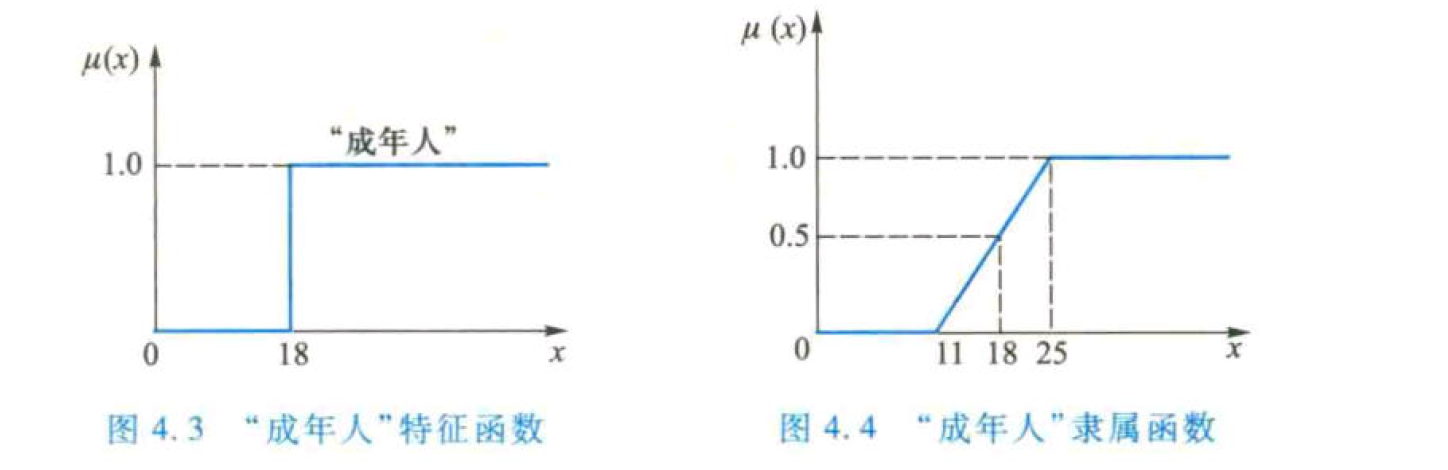• 序偶表示法
• 向量表示法
##### 隶属度函数
• 常见的隶属函数有正态分布、三角分布、梯形分布等。
• 隶属函数确定方法：模糊统计法, 专家经验法, 二元对比排序法, 基本概念扩充法

#### 模糊集合运算

• 交运算(intersection) $A \cap B$ :
• 并运算 (union) $A \cup B$ :
• 补运算 (complement) $\bar{A}$ 或者 $A^{C}$ :

• 代数积
• 代数和
• 有界和
• 有界积

#### 模糊关系与模糊关系的合成

• $\boldsymbol{A} 、 \boldsymbol{B}$: 模糊集合, 模糊关系用叉积(cartesian product)表示:$R: A \times B \rightarrow[0,1]$

• 积常用最小算子运算:$\mu_{A \times B}(a, b)=\min \left\{\mu_{A}(a), \mu_{B}(b)\right\}$

• $\boldsymbol{A} 、 \boldsymbol{B}$: 离散模糊集, 其隶属函数分别为:$\mu_{A}=\left[\mu_{A}\left(a_{1}\right), \mu_{A}\left(a_{2}\right), \cdots, \mu_{A}\left(a_{n}\right)\right], \quad \mu_{B}=\left[\mu_{B}\left(b_{1}\right), \mu_{B}\left(b_{2}\right), \cdots, \mu_{B}\left(b_{n}\right)\right]$则其叉积运算: $\quad \mu_{A \times B}(a, b)=\mu_{A}^{T} \circ \mu_{B}$

• 最大-最小合成法：写出矩阵乘积QR中的每个元素，然后将其中的乘积运算用取小运算代替，求和运算用取大运算代替。
• 最大-代数积合成法：写出矩阵乘积QR中的每个元素，然后将其中的求和运算用取大运算代替，而乘积运算不变。

#### 模糊推理

• 模糊知识表示 : 如果 （条件） → 则 （结论）

• 模糊规则：从条件论域到结论论域的模糊关系矩阵 R。通过条件模糊向量与模糊关系 R 的合成进行模糊推理，得到结论的模糊向量，然后采用“清晰化”方法将模糊结论转换为精确量。

• 对 IF A THEN B 类型的模糊规则的推理

• 若已知输入为$\boldsymbol{A}$, 则输出为$\boldsymbol{B}$; 若现在已知输入为$A^{\prime}$, 则输出$B^{\prime}$ 用合成规则求取$B^{\prime}=A^{\prime} \circ R$其中模糊关系 $R : \mu_{R}(x, y)=\min \left[\mu_{A}(x), \mu_{B}(y)\right]$
• 控制规则库的 $N$条规则有 $\boldsymbol{N}$个模糊关系:$R_{1}, R_{2}, \cdots, R_{n}$对于整个系统的全部控制规则所对应的模糊关系 $R$:

#### 模糊决策

• “模糊决策”(“模糊判决”、“解模糊”或“清晰化”）：由模糊推理得到的结论或者操作是一个模糊向量，转化为确定值的过程。
• 最大隶属度法
• 加权平均判决法

• 中位数法

#### ==例题==

1. 确定模糊关系R
1. 模糊推理
1. 模糊决策

​ 用最大隶属度法进行决策得风门开度为5。用加权平均判决法和中位数法进行决策得风门开度为4。

## 搜索求解

### 分类

• 盲目搜索：在不具有对特定问题的任何有关信息的条件下，按固定的步骤（依次或随机调用操作算子）进行的搜索。

• 启发式搜索：考虑特定问题领域可应用的知识，动态地确定调用操作算子的步骤，优先选择较适合的操作算子，尽量减少不必要的搜索，以求尽快地到达结束状态。

### 状态空间表示法

• 状态：表示系统状态、事实等叙述型知识的一组变量或数组：
• 操作：表示引起状态变化的过程型知识的一组关系或函数：
• 状态空间：利用状态变量和操作符号，表示系统或问题的有关知识的符号体系，状态空间是一个四元组：
• 求解路径：从$S_0$结点到$G$结点的路径。
• 状态空间解：一个有限的操作算子序列。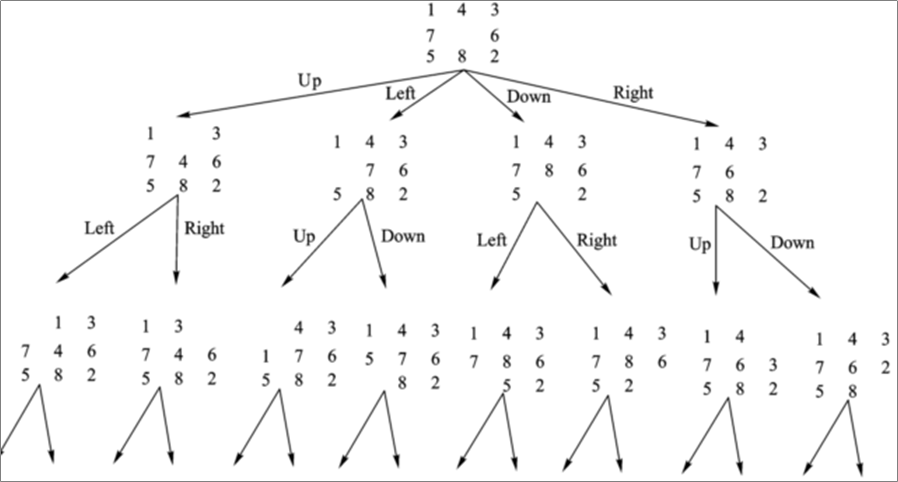### 回溯法

#### 回溯法原理

##### 步骤
• 用未处理状态表（NPS）使算法能返回（回溯）到其中任一状态。
• 用一张“死胡同”状态表（NSS）来避免算法重新搜索无解的路径。
• 在PS 表中记录当前搜索路径的状态，当满足目的时可以将它作为结果返回。
• 为避免陷入死循环必须对新生成的子状态进行检查，看它是否在该三张表中 。

##### 回溯法解决的问题

• 组合问题：N个数里面按一定规则找出k个数的集合
• 切割问题：一个字符串按一定规则有几种切割方式
• 子集问题：一个N个数的集合里有多少符合条件的子集
• 排列问题：N个数按一定规则全排列，有几种排列方式
• 棋盘问题：N皇后，解数独等等

##### 回溯法模板

void backtracking(参数) {
if (终止条件) {
存放结果;
return;
}

for (选择：本层集合中元素（树中节点孩子的数量就是集合的大小）) {
处理节点;
backtracking(路径，选择列表); // 递归
回溯，撤销处理结果
}
}


#### 宽度优先搜索（BFS）

• open表（NPS表)：已经生成出来但其子状态未被搜索的状态。
• closed表（ PS表和NSS表的合并）：记录了已被生成扩展过的状态。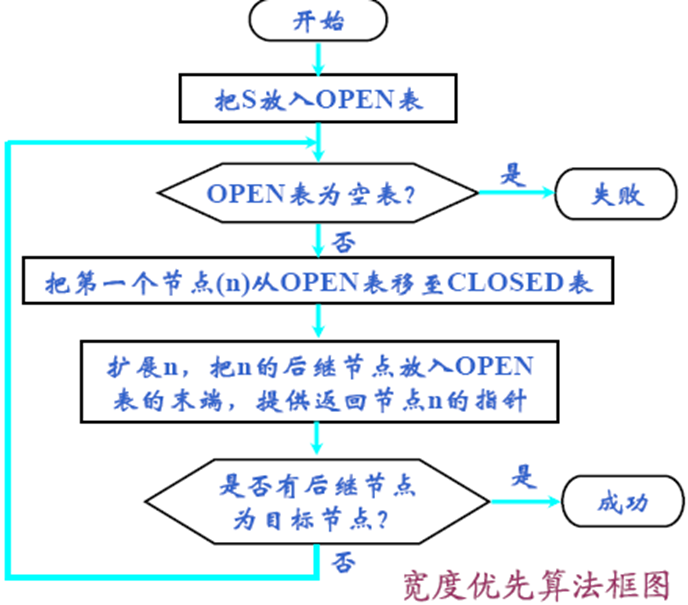#### 深度优先搜索（DFS）

• 防止搜索过程沿着无益的路径扩展下去，往往给出一个节点扩展的最大深度——深度界限；
• 与宽度优先搜索算法最根本的不同：将扩展的后继节点放在OPEN表的前端。
• 深度优先搜索算法的OPEN表后进先出。

### 启发式搜索

#### 启发式信息

• 启发式图搜索策略（利用启发信息的搜索方法）的特点：重排OPEN表，选择最有希望的节点加以扩展。

1. 陈述性启发信息：用于更准确、更精炼地描述状态
2. 过程性启发信息：用于构造操作算子
3. 控制性启发信息：表示控制策略的知识

1. 用于扩展节点的选择，即用于决定应先扩展哪一个节点，以免盲目扩展。
2. 用于生成节点的选择，即用于决定要生成哪些后继节点，以免盲目生成过多无用的节点。
3. 用于删除节点的选择，即用于决定删除哪些无用节点，以免造成进一步的时空浪费。

#### 估价函数

• A 搜索算法：使用了估价函数 $f$的最佳优先搜索。

### ==例题==

#### 积木问题

##### 要求

1、被搬动积木顶必为空

2、若Y是积木，Y顶部也必须为空

3、同状态下操作算子运用次数不得多于1次

##### 过程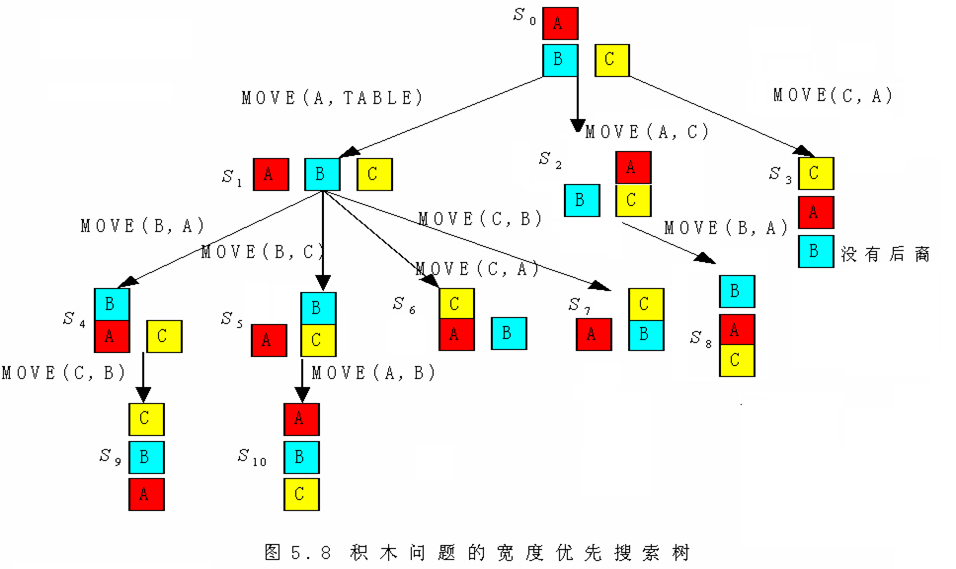Open表：S6， S7 ， S8 ，S9 ， S10

Closed表：S0 ，S1 ，S2，S3 ，S4，S5

#### 卒子穿阵（迷宫）

1 1 0 0 0
2 0 0 1 0
3 0 1 0 0
4 1 0 0 0
##### 步骤

（1,3） - > (1,4) 大于（1,4）直接出发

• 从（1，n）出发，为不同出发点，（1，n）的下一个状态只能为（2，n）
• （n,m）（n>1）下一个状态优先级依次为： {（n+1,m）,(n,m-1),(n,m+1)}(不能后退)
• 遇到边缘和敌兵（1）状态为死
• 最大深度为5
• 注：如过求全部路径，直接在（1，n）状态之间平移转换。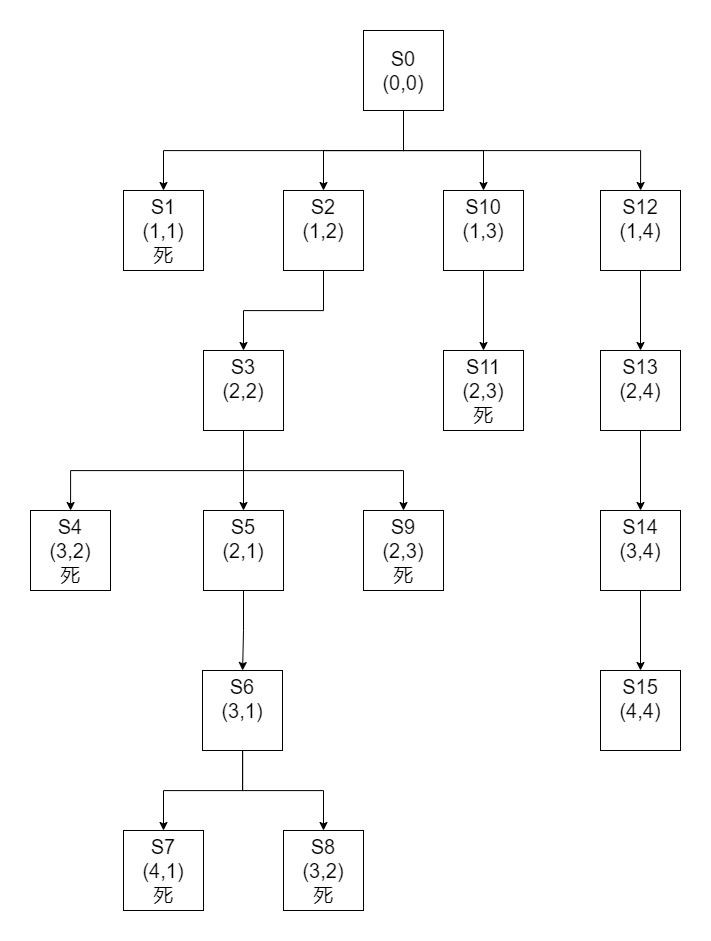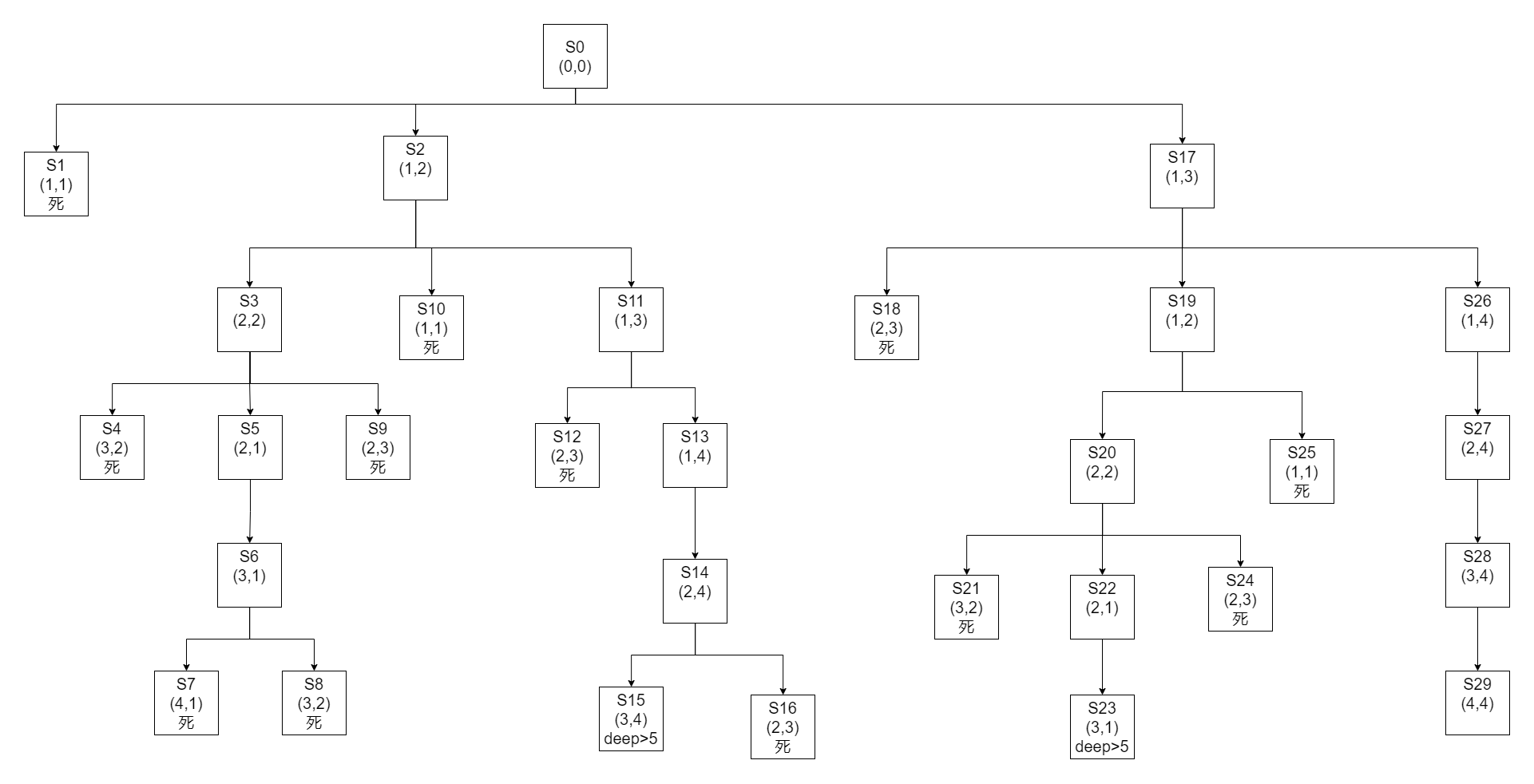maybe：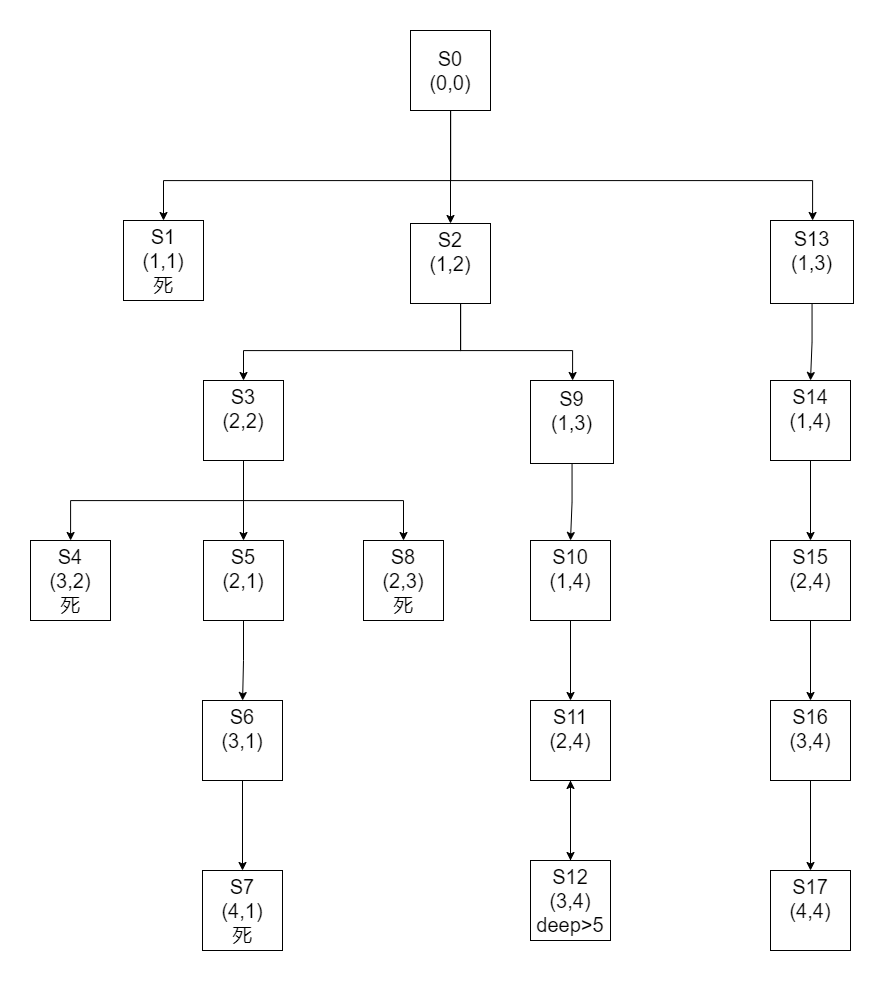目录